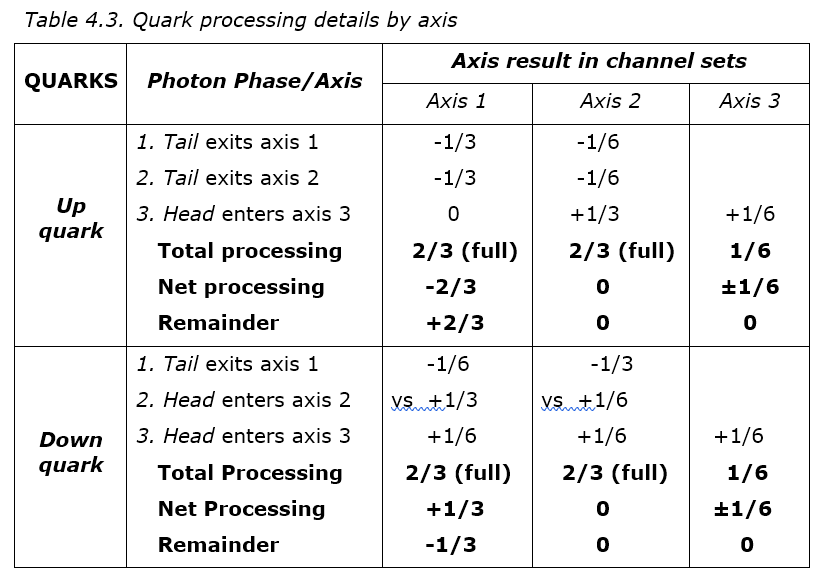# QR4.4.3 Quark Phases

As for an electron, a three-way interaction of extreme photons has phase options that can be expressed in photon head-tail terms. Again, a tail-tail-tail meet isn’t possible as it implies a prior head-head-head event. The phase options are:

1. Head-head-head. Three sets of photon heads meeting at equal angles in a node allocate processing equally so each axis is only partly filled. There are free channels that let other entities in, so the result isn’t stable.

2. Head-tail-tail. In this case, two photon rays leave a node as another arrives, as shown in Figure 4.10a, and this is proposed to be an up quark.

3. Head-head-tail. In this case one ray has passed through the node as the other two arrive, as shown in Figure 4.10b, and this is proposed to be a down quark.

Figure 4.10 shows the proposed up and down quark structures. As photons compete for channels on a first-come-first-served basis, a three-way meeting raises the issue of interaction order. If a photon head entering a node meets a photon tail leaving it, the tail must start before the head or it would be a head, giving the rule that tails fill channels first. Using this rule, Table 4.3 gives the expected axis bandwidth result as before, except now there are three axes not one and each fills at two-thirds not one. Again, the total processing defines stability, the mass is the net processing and the charge is the net remainder.The details are:

1. Up quark. If two extreme photon rays leave a node as another arrives, the tails first fill axis 1, giving a plus two-thirds charge remainder on this charge axis. The remaining tail photons then combine with later arriving heads to fill a neutral axis, as the remainders cancel. The remaining head photons partly fill the third free axis to a sixth instead of its maximum value of two-thirds. The result has a two-thirds charge and is stable on two axes but has spare photons in the third axis.

2. Down quark. If one ray has passed through a node as the other two arrive, the tail photons first cancel opposing heads to fill a neutral axis as the remainders cancel. Then the heads and the remaining tails fill a charge axis with a minus third charge left over. This again leaves a third free axis partly filled to a sixth instead of two thirds. The result has a minus third charge and is again stable on two axes with again spare photons in the third axis.

This result is interesting because it gives the correct third charges for quarks which no other model does. While the standard model allocates one-third charges to quarks after the fact, this model derives them. It predicts that quarks occupy one node like leptons but only fill two of the three collision axes.

To sum up, the three-axis structure derived for quarks is:

1. Charge axis. Fills with quark charge of up quark + and down quark –.

2. Neutral axis. Fills as heads and tails cancel with no remainder.

3. Free axis. Remaining one sixth of head photons partly fills this axis.